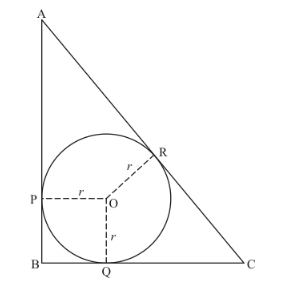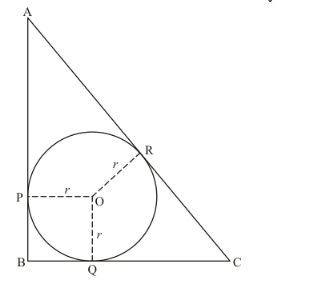# In the given figure, ABC is a right triangle right-angled at

Question:

In the given figure, ABC is a right triangle right-angled at B such that BC = 6 cm and AB = 8 cm. Find the radius of its incircle.Solution:

From the property of tangents we know that the length of two tangents drawn to a circle from the same external point will be equal. Therefore, we have

BQ = BP

Let us denote BP and BQ by x

AP = AR

Let us denote AP and AR by y

RC = QC

Let us denote RC and RQ by z

We have been given thatis a right triangle and BC = 6 cm and AB = 8 cm. let us find out AC using Pythagoras theorem. We have,

$A C^{2}=A B^{2}+B C^{2}$

$A C^{2}=6^{2}+8^{2}$

$A C^{2}=36+64$

$A C^{2}=100$

$A C=\sqrt{100}$

$A C=10$Consider the perimeter of the given triangle. We have,

AB + BC + AC = 8 + 6 + 10

AB + BC + AC = 24

Looking at the figure, we can rewrite it as,

AP + PB + BQ + QC + AR + RC = 24

Let us replace the sides with the respective x, y and z which we have decided to use.

$y+x+x+z+y+z=24$

$2 x+2 y+2 z=24$

$2(x+y+z)=24$

$x+y+z=12$.....................(1)

Now, consider the side AC of the triangle.

AC = 10

Looking at the figure we can say,

AR + RC = 10

y + z = 10 …… (2)

Now let us subtract equation (2) from equation (1). We have,

x + y + z = 12

y + z = 10

After subtracting we get,

x = 2

That is,

BQ = 2, and

BP = 2

Now consider the quadrilateral BPOQ. We have,

BP = BQ (since length of two tangents drawn to a circle from the same external point are equal)

Also,

PO = OQ (radii of the same circle)

It is given that.

From the property of tangents, we know that the tangent will be at right angle to the radius of the circle at the point of contact. Therefore,

$\angle O P B=90^{\circ}$

$\angle O Q B=90^{\circ}$

We know that sum of all angles of a quadrilateral will be equal to. Therefore,

$\angle P B Q+\angle O P B+\angle O Q B+\angle P O Q=360^{\circ}$

$90^{\circ}+90^{\circ}+90^{\circ}+\angle P O Q=360^{\circ}$

$270^{\circ}+\angle P O Q=360^{\circ}$

$\angle P O Q=90^{\circ}$

Since all the angles of the quadrilateral are equal toand the adjacent sides also equal, this quadrilateral is a square. Therefore, all sides will be equal. We have found out that,

BP = 2 cm